# Precalculus : Graph a Hyperbola

## Example Questions

### Example Question #3 : Hyperbolas

What is the equation of the conic section graphed below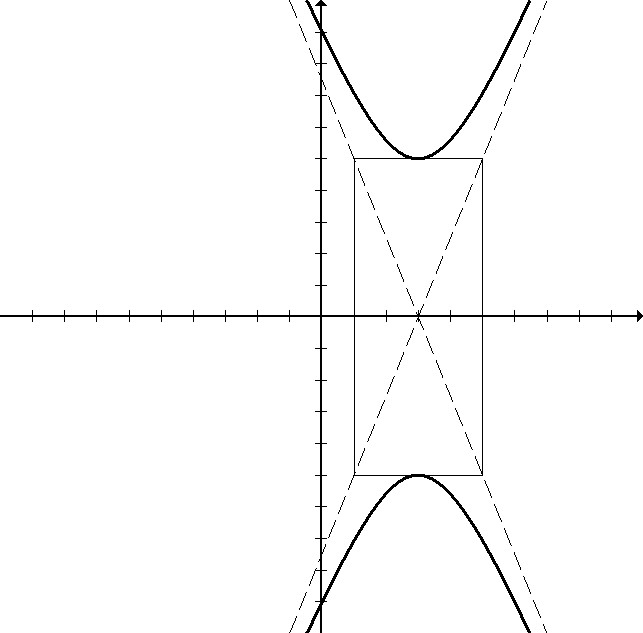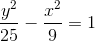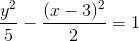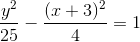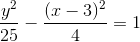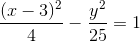Explanation:

The hyperbola pictured is centered at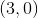, meaning that the equation has a horizontal shift. The equation must have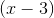rather than just x. The hyperbola opens up and down, so the equation must be the y term minus the x term. The hyperbola is drawn according to the box going up/down 5 and left/right 2, so the y term must beor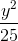, and the x term must be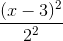or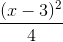.

### Example Question #4 : Hyperbolas

How can this graph be changed to be the graph of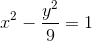?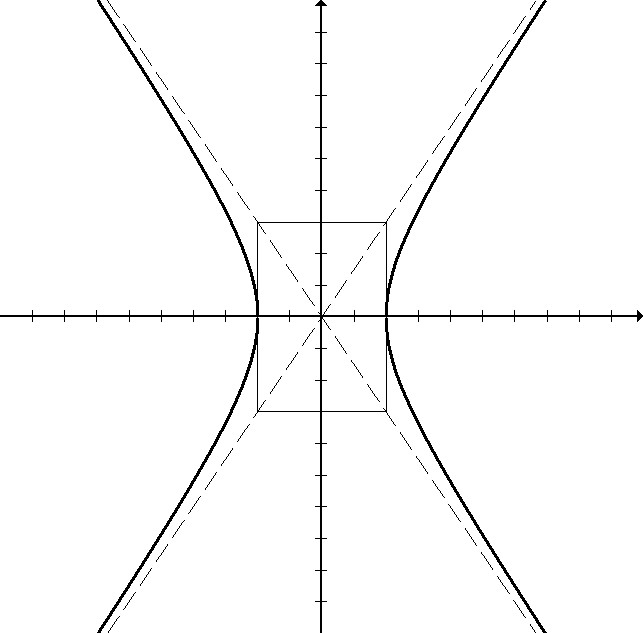The center box should extend up toand down to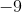, stretching the graph.

The-intercepts should be at the pointsand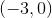.

The graph should be an ellipse and not a hyperbola.

The-intercepts should be at the points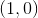and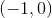.

The graph should have-intercepts and not-intercepts.

The-intercepts should be at the pointsand.

Explanation:

This equation should be thought of as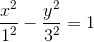.

This means that the hyperbola will be determined by a box with x-intercepts at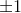and y-intercepts at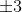.

The hyperbola was incorrectly drawn with the intercepts at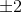instead.

### Example Question #5 : Hyperbolas

Which of the following would NOT be true of the graph for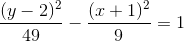?

The graph is centered at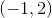.

The graph never intersects with the-axis.

The graph never intersects with the-axis.

The graph opens up and down.

All of these statements are true.

The graph never intersects with the-axis.

Explanation:

The graph should look like this: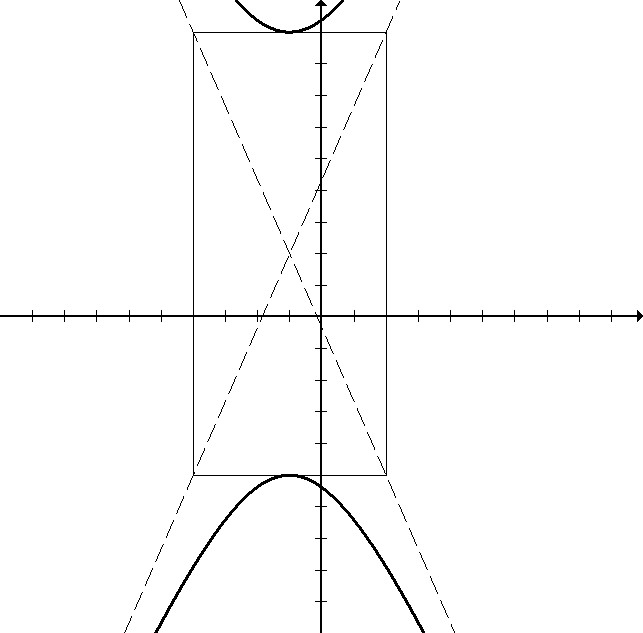### Example Question #6 : Hyperbolas

Which of these equations produce this graph, rotated 90 degrees?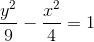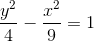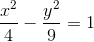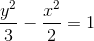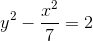Explanation:

Rotated 90 degrees, this graph would be opening up and down instead of left and right, so the equation will have the y term minus the x term.

The box that the hyperbola is drawn around will also rotate. It will now be up/down 2 and left/right 3.

This makes the correct equation.

### All Precalculus Resources